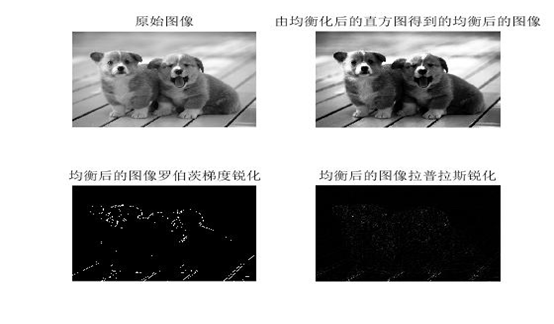# 图像处理程序

## MATLAB一些简单的图像处理程序

2016-10-10 18:18:48 yangkunpengD 阅读数 9127
• ###### 实现自己的Prisma（基于QT和OpenCV实现Android平台上基于AI的图像处理APP)

本课主要内容是基于QT和OpenCV实现... 重点为QT程序设计、OpenCV.DNN调用深度学习网络和编写完整的Android图像处理程序。 主要基于C++语言，基于QT/QML、OpenCV、torch等基础库和QTcreator。 </div>

28课时 337分钟 302人学习 禾路
免费试看

1

```I=imread('pout.tif');
figure,imshow(I);
[m,n]=size(I);
Inew=zeros(floor(m/2),n);
for i=1:m
for j=1:n
Inew(i,j)=I(2*i-1,j);
end
end
figure,imshow(uint8(Inew))```

2

```clc
clear
x=0:255;
y1=x;
figure,
plot(x,y1,'r')
hold on
y2=255:-1:0;
plot(x,y2,'b')
ylog=255*(log(x+1)/log(256));
plot(x,ylog,'g+')
yexp=255*x.^3/255^3;
plot(x,yexp,'y+')
c=255/255^3;
ynthroot=nthroot((x/c),3);
plot(x,ynthroot,'yo')```
3

```I=imread('moon.tif');
I=double(I);
[m,n]=size(I);
for i=1:m
for j=1:n
if I(i,j)<100
Inew(i,j)=3*I(i,j);
elseif I(i,j)>200
Inew(i,j)=0.8*I(i,j);
else
Inew(i,j)=I(i,j);
end
end
end
figure,imshow(Inew,[])```
4

```clear
clc
I=im2double(I);
[m,n]=size(I);
for i=1:m
for j=1:n
Inew(i,j)=I(i,j)^0.2;
end
end
figure,imshow(I,[])
figure,imshow(Inew,[])```
5

```clear
clc
I=im2double(I);
[m,n]=size(I);
%循环实现
tic
for i=1:m
for j=1:n
Inew(i,j)=255*log(I(i,j)+1)/log(256);
end
end
toc
%查找表
tic
x=0:255;
c=255/log(256);
y=c*log(x+1);
I_log=uint8(y(x+1));
figure,plot(x,y)
toc
figure,imshow(I_log,[])
figure,imshow(I,[])
figure,imshow(Inew,[])```

6

```I=imread('pout.tif');
figure,imshow(I)
[m,n]=size(I);
h=zeros(1,256);
for k=0:255
for i=1:m
for j=1:n
if(I(i,j)==k)
h(k+1)=h(k+1)+1;
end
end
end
end
figure,bar(h)```

```I=imread('pout.tif');
figure,imshow(I)
[m,n]=size(I);
h=zeros(1,256);
for i=1:m
for j=1:n
h(I(i,j)+1)=h(I(i,j)+1)+1;
end
end
figure,bar(h)```

```I=imread('pout.tif');
figure,imshow(I)
[m,n]=size(I);
h=zeros(1,256);
for i=1:m
for j=1:n
h(I(i,j)+1)=h(I(i,j)+1)+1;
end
end
figure,bar(h)
p=h/(m*n);
sum=0;
for i=1:256
sum=sum+p(i);
y(i)=round(sum*255);
end
for i=1:m
for j=1:n
Inew(i,j)=y(I(i,j)+1);
end
end

figure,imshow(unit8(Inew))```

7 邻域处理

```I=imread('pout.tif');
I=double(I);
%卷积模板的不同处理效果不同
%M=ones(3,3)/9;将一幅图模糊处理； M=[0 -1 0;-1 5 -1;0 -1 0];将一幅图锐化处理，改变中心位置的值有不同效果
%M=[1 1 1;0 0 0;-1 -1 -1];指出原始图像中水平边缘存在位置的图像；M=[1 0 -1;1 0 -1;1 0 -1];指出原始图像中垂直边缘存在位置的图像
M=ones(3,3)/9;
[m,n]=size(I);
Inew=zeros(m,n);
%从2开始到m-1忽略边界
for i=2:m-1
for j=2:n-1
Inew(i,j)=I(i-1,j-1)*M(1,1)+I(i-1,j)*M(1,2)+...
I(i-1,j+1)*M(1,3)+I(i,j-1)*M(2,1)+I(i,j)*M(2,2)+...
I(i,j+1)*M(2,3)+I(i+1,j-1)*M(3,1)+I(i+1,j)*M(3,2)+...
I(i+1,j+1)*M(3,3);
end
end
figure,imshow(uint8(Inew))```

## 遥感图像处理流程

2018-11-21 14:59:14 ctyqy2015301200079 阅读数 1968
• ###### 实现自己的Prisma（基于QT和OpenCV实现Android平台上基于AI的图像处理APP)

本课主要内容是基于QT和OpenCV实现... 重点为QT程序设计、OpenCV.DNN调用深度学习网络和编写完整的Android图像处理程序。 主要基于C++语言，基于QT/QML、OpenCV、torch等基础库和QTcreator。 </div>

28课时 337分钟 302人学习 禾路
免费试看

1．降噪处理

（1）除周期性噪声和尖锐性噪声图1 消除噪声前图2 消除噪声后

（2）除坏线和条带图3 去条纹前图4 去条纹后图5 去条带前图6 去条带后

2．薄云处理

3．阴影处理

1．图像配准

（1）影像对栅格图像的配准图7 图像配准前图8 图像配准后

（2）影像对矢量图形的配准

2．几何粗纠正

3．几何精纠正

（1）图像对图像的纠正

（2）图像对地图（栅格或矢量）图9 参考地形图图10 待纠正影像（3）图像对已知坐标点（地面控制点）

4．正射纠正1．彩色合成图13真彩色合成( TM321)图14 假彩色合成(TM432)

2．直方图变换图15 直方图拉伸前（原图偏暗）图16 直方图拉伸后图17 直方图拉伸前（原图对比度不强）图18 直方图拉伸后（线性拉伸）

3．密度分割图19 原始图像图20 密度分割图像

4．灰度颠倒图21 灰度颠倒前图22 灰度颠倒后

5．图像间运算图23 原始图像图24 NDVI植被指数图像

6．邻域增强图25 原始图像图26 拉普拉斯滤波图像（5×5）

7．主成分分析图27 第一主成分图28 第二主成分图29 第三主成分图30 第四主成分图31第五主成分图32 第六主成分

8．K-T变换图33 第一主分量（亮度）图34 第二主分量（绿度）图35第三主分量

9．图像融合图36 多光谱影像图 37高分辨率影像1．按ROI裁剪2．按文件裁剪

3．按地图裁剪1．图像镶嵌图42镶嵌左影像图43 镶嵌右影像2．影像匀色图45 匀色前影像图46 匀色后影像

1．目视判读2．图像分类

（1）监督分类图48 原图像图49 监督分类图像

（2）非监督分类

（3）其他分类方

## 基于MATLAB的图像处理程序（…

2016-05-23 10:34:10 wangxiaofei558 阅读数 11419
• ###### 实现自己的Prisma（基于QT和OpenCV实现Android平台上基于AI的图像处理APP)

本课主要内容是基于QT和OpenCV实现... 重点为QT程序设计、OpenCV.DNN调用深度学习网络和编写完整的Android图像处理程序。 主要基于C++语言，基于QT/QML、OpenCV、torch等基础库和QTcreator。 </div>

28课时 337分钟 302人学习 禾路
免费试看

1.     图像变换

clear all

N=100;

f=zeros(50,50);          %产生一个50*50的全0数组

f(15:35,23:28)=1;

%定义图像数组，从15行到35行，23列到28列附值为1，为白色，其他区域为黑色

figure(1)                %创建窗口的图形对象，句柄为1

imshow(f,'notruesize')     %显示图像f

F=fft2(f,N,N);           %在二维傅立叶变换前把f截断或者添加0，使其成为N*N的数组

F2=fftshift(abs(F));       %把傅立叶变换的零频率部分移到频谱的中间

figure(2)

x=1:N;y=1:N;           %定义x和y的范围

mesh(x,y,F2(x,y));colormap(gray);colorbar

%绘制立体网状图，将图形对象的色度改为灰度图像，colorbar给坐标轴添加色彩条

%构建一个类似于figure(1)的矩形函数

N=200;

f=zeros(100,100);

f(30:70,45:55)=1;

%定义图像数组，从30行到70行，45列到55列附值为1，为白色，其他区域为黑色

imshow(f,'notruesize');

%然后对f进行二维快速傅立叶变换：以下列出你自己编写的代码…

N=200;

f=zeros(100,100);

f(30:70,45:55)=1;

imshow(f,'notruesize');

title('原始图像');

F=fft2(f,N,N);                 %对图像f进行二维快速傅立叶变换

grid on                       %打开网格线

axis on                       %打开坐标轴

imshow(F,[-1,5],'notruesize');     %显示傅立叶变换后的图像，图像数据的值域为[-1,5]

x=1:N;y=1:N;

title('二维快速傅立叶变换后的图像');

mesh(abs(F));                 %绘制F的频谱图

title('傅立叶变换后的频谱图');

%然后对上述二维快速傅立叶变换提高分辨率：

要提高二维快速傅立叶变换的分辨率，在采样率一定的情况下，增大采

N=300;

f=zeros(100,100);

f(30:70,45:55)=1;

figure(1)

imshow(f,'notruesize');

title('原始图像');

F=fft2(f,N,N);

axis on

figure(2)

imshow(F,[-1,5],'notruesize');

title('二维快速傅立叶变换后的图像');

x=1:N;y=1:N;

figure(3)

mesh(abs(F));

title('傅立叶变换后的频谱图');

N=400;

f=zeros(100,100);

f(30:70,45:55)=1;

figure(1)

imshow(f,'notruesize');

title('原始图像');

F=fft2(f,N,N);

axis on

figure(2)

imshow(F,[-1,5],'notruesize');

title('二维快速傅立叶变换后的图像');

x=1:N;y=1:N;

figure(3)

mesh(abs(F));

title('傅立叶变换后的频谱图');

�系数移动（使用函数fftshift）

N=200;

f=zeros(100,100);

f(30:70,45:55)=1;

figure(1)

imshow(f,'notruesize');

title('原始图像');

F=fft2(f,N,N);

axis on

figure(2)

imshow(F,[-1,5],'notruesize');

title('二维快速傅立叶变换后的图像');

x=1:N;y=1:N;

figure(3)

mesh(fftshift(abs(F)));

%把零频率部分移到频谱的中间

title('傅立叶变换后的频谱图');

%滤波器频率响应

x=1:N;y=1:N;

mesh(x,y,F2(x,y));colormap(gray);colorbar

%绘制立体网状图，将图形对象的色度改为灰度图像，colorbar给坐标轴添加色彩条

1》对f进行二维快速傅立叶变换

（请自己运行查看）

2》对上述二维快速傅立叶变换提高分辨率

N=300时：                         N=400时：

N=800时：                              N=1000时：

（请自己运行查看）

从傅立叶变换的频谱图中可以看出，提高分辨率以后，其边缘更加平滑，锯齿状明显减弱。但其傅立叶变换后的图像没有明显改变。

3》DC系数移动

4》滤波器频率响应

（请自己运行查看）

2. 图像增强

subplot(121)

imshow(I);

title('原始图像');

subplot(122)

imhist(I,64)

%绘制图像的直方图，n=64为灰度图像灰度级，若I为灰度图像，默认n=256；

title('图像的直方图');

（请自己运行查看）

n=256时：

（请自己运行查看）

J=histeq(I);

%将灰度图像转换成具有64（默认）个离散灰度级的灰度图像

imshow(I)

title('原始图像')

figure,imshow(J)

title('直方图均衡化后的图像')

figure(1)

subplot(121);imhist(I,64)

title('原始图像的直方图')

subplot(122);imhist(J,64)

title('均衡化的直方图')

（请自己运行查看）

figure,imshow(I);

title('原始图像');

hgram=50:2:250;       %规定化函数

J=histeq(I,hgram);

figure,imshow(J);

title('直方图规定化后的图像');

figure,imhist(I,64);

title('原始图像的直方图');

figure,imhist(J,64);

title('直方图规定化后的直方图');

（请自己运行查看）

hgram=50:1:250;                    hgram=50:5:250;

3. 图像重建

（1）联立方程法（也称矩阵法）；

（2）逆投影法；

（3）付立叶变换法；

（4）滤波──逆投影法（也称卷积法）；

（5）逐次逼近法。

P=phantom(256);   %用phantom函数产生Sheep-Logan的大脑图，n为图像p中的行列数，默认为256

imshow(P)

title('原始图像')

%以下为三种不同角度的投影模式

figure,imagesc(theta3,xp,R3);colormap(hot);colorbar;

xlabel('theta');ylabel('xprime');     %定义坐标轴

figure,imshow(I1)

figure,imshow(I2)

figure,imshow(I3)

（请自己运行查看）

4. 滤波

4.1 目的

4.2 使用设备

PC兼容机一台，操作系统为Windows2000（或Windows98，WindowsXP，以下默认为Windows2000）

4.3 使用滤波对图像进行增强

4.3.1 线性滤波（邻域平均）

imshow(I)

title('原始图像')

J=imnoise(I,'salt & pepper');    %添加盐椒噪声，噪声密度为默认值0.05

figure,imshow(J)

title('添加盐椒噪声后的图像')

K1=filter2(fspecial('average',3),J)/255;   %应用3×3邻域窗口法

figure,imshow(K1)

title('3×3窗的邻域平均滤波图像')

K2=filter2(fspecial('average',7),J)/255;   %应用7×7邻域窗口法

figure,imshow(K2)

title('7×7窗的邻域平均滤波图像')

K3=filter2(fspecial('average',9),J)/255;   %应用9×9邻域窗口法

figure,imshow(K3)

title('9×9窗的邻域平均滤波图像')

K4=filter2(fspecial('average',11),J)/255;   %应用11×11邻域窗口法

figure,imshow(K4)

title('11×11窗的邻域平均滤波图像')

（请自己运行查看）

4.3.2 中值滤波

imshow(I)

title('原始图像')

J=imnoise(I,'salt & pepper',0.02);  %添加盐椒噪声，噪声密度为0.02

figure,imshow(J)

title('添加盐椒噪声后的图像')

K1=medfilt2(J);               %在默认的3×3的邻域窗中进行中值滤波

figure,imshow(K1)

title('默认的3×3的邻域窗的中值滤波图像')

K2=medfilt2(J,[5 5]);           %在5×5的邻域窗中进行中值滤波

figure,imshow(K2)

title('5×5的邻域窗的中值滤波图像')

（请自己运行查看）

4.3.3 锐化滤波

Laplacian算子是线性二次微分算子，其格式为：h = fspecial('laplacian', alpha)，返回一个3×3的滤波器来近似二维Laplacian算子的形状，参数alpha决定了Laplacian算子的形状，alpha的取值范围为0.0~1.0，默认的值为0.2。

%应用Laplacian算子对图像进行锐化

imshow(I)

title('原始图像')

H=fspecial('laplacian');

%应用laplacian算子滤波锐化图像

laplacianH=filter2(H,I);

figure,imshow(laplacianH)

title('laplacian算子锐化后的图像')

（请自己运行查看）

5. 边缘检测

BW=edge(I,'sobel',thresh,direction)   根据指定的敏感阈值thresh用Sobel算子对图像进行边缘检测，edge函数忽略了所有小于阈值的边缘，如果没有指定阈值thresh或为空，函数自动选择参数值，direction指定Sobel算子边缘检测的方向，其参数值为'horizontal'，'vertical'或'both'（默认）。

imshow(I)

title('原始图像')

BW=edge(I,'sobel');

%以自动域值选择法对图像进行Sobel算子边缘检测

figure,imshow(BW);

title('自动域值的Sobel算子边缘检测')

[BW,thresh]=edge(I,'sobel');

%返回当前Sobel算子边缘检测的阈值

disp('sobel算子自动选择的阈值为：')

disp(thresh)

BW1=edge(I,'sobel',0.02,'horizontal');

%以域值为0.02水平方向对图像进行Sobel算子边缘检测

figure,imshow(BW1)

title('域值为0.02的水平方向的sobel算子检测')

BW2=edge(I,'sobel',0.02,'vertical');

%以域值为0.02垂直方向对图像进行Sobel算子边缘检测

figure,imshow(BW2)

title('域值为0.02的垂直方向的sobel算子检测')

BW3=edge(I,'sobel',0.05,'horizontal');

%以域值为0.05水平方向对图像进行Sobel算子边缘检测

figure,imshow(BW3)

title('域值为0.05的水平方向的sobel算子检测')

BW4=edge(I,'sobel',0.05,'vertical');

%以域值为0.05垂直方向对图像进行Sobel算子边缘检测

figure,imshow(BW4)

title('域值为0.05的垂直方向的sobel算子检测')

（请自己运行查看）

sobel算子自动选择的阈值为：0.1433

BW=edge(I,'prewitt',thresh,direction) 根据指定的敏感阈值thresh用Prewitt算子对图像进行边缘检测。

imshow(I)

title('原始图像')

BW=edge(I,'prewitt');

%以自动域值选择法对图像进行Prewitt算子边缘检测

figure,imshow(BW);

title('自动域值的prewitt算子边缘检测')

[BW,thresh]=edge(I,'prewitt');

%返回当前Prewitt算子边缘检测的阈值

disp('prewitt算子自动选择的阈值为：')

disp(thresh)

BW1=edge(I,'prewitt',0.02,'horizontal');

%以域值为0.02水平方向对图像进行Prewitt算子边缘检测

figure,imshow(BW1)

title('域值为0.02的水平方向的prewitt算子检测')

BW2=edge(I,'prewitt',0.02,'vertical');

%以域值为0.02垂直方向对图像进行Prewitt算子边缘检测

figure,imshow(BW2)

title('域值为0.02的垂直方向的prewitt算子检测')

BW3=edge(I,'prewitt',0.05,'horizontal');

%以域值为0.05水平方向对图像进行Prewitt算子边缘检测

figure,imshow(BW3)

title('域值为0.05的水平方向的prewitt算子检测')

BW4=edge(I,'prewitt',0.05,'vertical');

%以域值为0.05垂直方向对图像进行Prewitt算子边缘检测

figure,imshow(BW4)

title('域值为0.05的垂直方向的prewitt算子检测')

（请自己运行查看）

prewitt算子自动选择的阈值为：0.1399

6. 图像压缩

图像压缩是指以较少的比特有损或无损地表示原来的像素矩阵的技术，也称图像编码。

从压缩编码算法原理上可以分类为：

(1)无损压缩编码种类：哈夫曼编码、算术编码、行程编码、Lempel zev编码

(2)有损压缩编码种类：

(3)混合编码 ?JBIG，H261，JPEG，MPEG等技术标准

DCT先将整体图像分成N×N像素块（一般N=8 ，即64个像素块），再对N×N块像素逐一进行DCT变换。由于大多数图像高频分量较小，相应于图像高频成分的失真不太敏感，可以用更粗的量化，在保证所要求的图质下，舍弃某些次要信息。

imshow(I);

title('原始图像')

disp('原始图像大小：')

whos('I')

I=im2double(I);

%图像类型存储转换，将图像矩阵转换成双精度类型

T=dctmtx(8);

%离散余弦变换矩阵

B=blkproc(I,[8 8],'P1*x*P2',T,T');

mask=[1 1 1 1 0 0 0 0

1 1 1 0 0 0 0 0

1 1 0 0 0 0 0 0

1 0 0 0 0 0 0 0

0 0 0 0 0 0 0 0

0 0 0 0 0 0 0 0

0 0 0 0 0 0 0 0

0 0 0 0 0 0 0 0];

I2=blkproc(B2,[8 8],'P1*x*P2',T',T);

figure,imshow(I2);

title('压缩后的图像')

disp('压缩图像的大小：')

whos('I2')

（请自己运行查看）

Name        Size              Bytes  Class    Attributes

256x256            65536  uint8

Name        Size              Bytes  Class     Attributes

I2        256x256            524288  double

clear all

imshow(I);                   %显示图像

title('原始图像')

disp('原始图像I的大小:');

whos('I')

I=im2double(I);

[c,s]=wavedec2(I,2,'bior3.7');     %对图像用小波进行层分解

cal=appcoef2(c,s,'bior3.7',1);     %提取小波分解结构中的一层的低频系数和高频系数

ch1=detcoef2('h',c,s,1);          %提取二维水平方向细节系数

cv1=detcoef2('v',c,s,1);          %提取二维垂直方向细节系数

cd1=detcoef2('d',c,s,1);          %提取二维对角线方向细节系数

ca1=appcoef2(c,s,'bior3.7',1);     %保留小波分解第一层低频信息

ca1=wcodemat(ca1,440,'mat',0);   %首先对第一层信息进行量化编码

ca1=0.5*ca1;                  %改变图像高度

figure,image(ca1);              %显示压缩后的图象

title('第一次压缩后的图像')

disp('第一次压缩图像的大小为：')

whos('ca1')

ca2=appcoef2(c,s,'bior3.7',2);     %保留小波分解第二层低频信息进行压缩

ca2=wcodemat(ca2,440,'mat',0);   %首先对第二层信息进行量化编码

ca2=0.25*ca2;                 %改变图像高度

figure,image(ca2);              %显示压缩后的图象

title('第二次压缩后的图像')

disp('第二次压缩图像的大小为：')

whos('ca2')

（请自己运行查看）

Name      Size                   Bytes  Class

256x256                  65536  uint8 array

Grand total is 65536 elements using 65536 bytes

Name      Size                   Bytes  Class

ca1     135x135                 145800  double array

Grand total is 18225 elements using 145800 bytes

Name      Size                   Bytes  Class

ca2      75x75                   45000  double array

Grand total is 5625 elements using 45000 bytes

## 图像处理分类、一般流程与算法

2017-06-08 10:07:00 weixin_30788731 阅读数 546
• ###### 实现自己的Prisma（基于QT和OpenCV实现Android平台上基于AI的图像处理APP)

本课主要内容是基于QT和OpenCV实现... 重点为QT程序设计、OpenCV.DNN调用深度学习网络和编写完整的Android图像处理程序。 主要基于C++语言，基于QT/QML、OpenCV、torch等基础库和QTcreator。 </div>

28课时 337分钟 302人学习 禾路
免费试看1. 数字图像处理-概述

1950s：二维图像分析和识别，如光学字符识别，工件表面、显微图片和航空图片的分析和解释等。是模式识别的重要内容。

1960s：MIT的Roberts通过计算机程序从数字图像中提取出诸如立方体、楔形体、棱柱体等多面体的三维结构，并对物体形状及物体的空间关系进行描述．这项研究开创了以理解三维场景为目的的三维计算机视觉的研究．Roberts对积木世界的创造性研究给人们以极大的启发，许多人相信，一旦由白色积木玩具组成的三维世界可以被理解，则可以推广到理解更复杂的三维场景．

1970s：出现了一些视觉应用系统．

-70年代中期，麻省理工学院(MIT)人工智能(AI)实验室正式开设“计算机视觉” ( Machine Vision) 课程，由B．K．P．Horn教授讲授．

-David Marr教授于1973年应邀在MIT AI 实验室领导一个以博士生为主体的研究小组，1977年提出了不同于“积木世界”分析方法的计算视觉理论.

1980s：Marr理论成为计算机视觉研究领域中的一个十分重要的理论框架．(ICCV, Marr奖)

计算机视觉获得蓬勃发展，新概念、新方法、新理论、新应用不断涌现，比如，基于感知特征群的物体识别理论框架，主动视觉理论框架，视觉集成理论框架等．

Marr模型

80年代初，Marr首次从信息处理的角度综合了图像处理、心理物理学、神经生理学及临床神经病理学的研究成果，提出了第一个较完备的视觉系统框架。

Marr将系统分为基元图、2.5维图（部分的、不完整的三维信息，缺少深度信息）和三维模型三个层次来表达视觉信息的处理过程，而每层的表达将适当的信息明朗化。2.5维图：使表面朝向的信息明朗化，如场景可见部分的深度、法线方向、轮廓等。

- 图像处理 (Image Processing) 图像处理通常是把一幅图像变换成另外一幅图像，也就是说，图像处理系统的输入是图像，输出仍然是图像，信息恢复任务则留给人来完成。

- 计算机图形学 (Computer Graphics) 通过几何基元，如线、圆和自由曲面等，来生成图像，属于图像综合，它在可视化（Visualization）和虚拟现实(Virtual Reality）中起着很重要的作用．计算机视觉正好是解决相反的问题，即从图像中估计几何基元和其它特征，属于图像分析．

- 模式识别 (Pattern Recognition) 研究分类问题，确定符号、图画、物体等输入对象的类别．强调一类事物区别于其它事物所具有的共同特征。一般不关心三维世界的恢复问题。

- 人工智能 (Artificial Intelligence) 涉及到智能系统的设计和智能计算的研究．在经过图像处理和图像特征提取过程后，接下来要用人工智能方法对场景特征进行表示，并分析和理解场景．

- 媒体计算 (Multimedia Computing) 文字\图形\图像\动画\视频\音频等各类感觉媒体的共性基础计算理论、计算方法，以及媒体系统实现技术。以实现下一代计算机能听、能看、会说、会学习为目标。

- 认知科学与神经科学 (Cognitive science and Neuroscience) 将人类视觉作为主要的研究对象．计算机视觉中已有的许多方法与人类视觉极为相似．许多计算机视觉研究者对研究人类视觉计算模型比研究计算机视觉系统更感兴趣，希望计算机视觉更加自然化，更加接近生物视觉

-图像处理：图像采集、储存；图像重建；图像变换、增强、恢复、校正；图像(视频)压缩编码。

-图像分析：边缘检测、图像分割；目标表达、描述；目标颜色、形状、纹理、空间和运动分析；目标检测、识别。

-图像理解：图像配准、融合；3-D表示、建模、场景恢复；图像感知、解释、推理；基于内容的图像和视频检索。

-工业领域（生产装配、质量检验）

-机器人（星球探测机器人）

-遥感图像分析（植被分析）

-医学图像分析（骨骼定位）

-安全鉴别、监视与跟踪（门禁系统、视频监控）

-国防系统（目标自动识别与目标跟踪）

-图像与视频检索（基于内容的检索）

-文物保护（数字博物馆）

-其他（游戏、动画、体育、人机交互）

-......

Learning OpenCV：http://www.cnblogs.com/2008nmj/p/6133737.html

## 数字图像处理--图像增强MATLAB程序

2018-08-20 08:42:43 iefenghao 阅读数 9840
• ###### 实现自己的Prisma（基于QT和OpenCV实现Android平台上基于AI的图像处理APP)

本课主要内容是基于QT和OpenCV实现... 重点为QT程序设计、OpenCV.DNN调用深度学习网络和编写完整的Android图像处理程序。 主要基于C++语言，基于QT/QML、OpenCV、torch等基础库和QTcreator。 </div>

28课时 337分钟 302人学习 禾路
免费试看

### 1 图像增强--灰度

``````//灰度
I=rgb2gray(P);
subplot(231);imshow(I,[]);title('原始图像');
subplot(234);imhist(I);title('由原始图像得到的直方图');
subplot(232);imshow(J);title('由新直方图得到的新图像');
K=histeq(I);
subplot(233);imshow(K);title('由均衡化后的直方图得到的均衡后的图像');
subplot(236);imhist(K);title('均衡化后的直方图');
``````

### 实验结果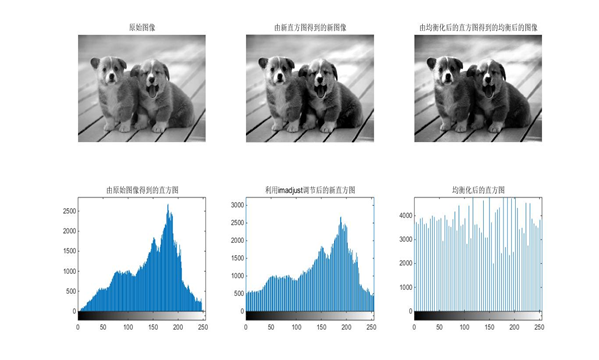### 2 图像增强--平滑

``````//平滑
I=rgb2gray(P);
subplot(221);imshow(I);title('原始图像');
I1=imnoise(I,'salt & pepper');
subplot(222);imshow(I1);title('加入椒盐噪声后的图像');
h1=ones(3,3)/9;
h2=ones(5,5)/25;
K1=imfilter(I1,h1);
K2=imfilter(I1,h2);
%subplot(334),imshow(I),title('原始图像');
%subplot(337),imshow(I),title('原始图像');
subplot(223),imshow(K1,[]),title('加入椒盐噪声后3*3平均滤波');
subplot(224),imshow(K2,[]),title('加入椒盐噪声后5*5平均滤波');

I2=imnoise(I,'gaussian',0,0.005);
figure;
subplot(221);imshow(I);title('原始图像');
subplot(222);imshow(I2);title('加入高斯噪声后的图像');
h1=ones(3,3)/9;
h2=ones(5,5)/25;
K1=imfilter(I2,h1);
K2=imfilter(I2,h2);
subplot(223),imshow(K1,[]),title('加入高斯噪声后3*3平均滤波');
subplot(224),imshow(K2,[]),title('加入高斯噪声后5*5平均滤波');

figure;
subplot(321);imshow(I1);title('加入椒盐噪声后的图像');
subplot(322);imshow(I2);title('加入高斯噪声后的图像');
J1=medfilt2(I1);
J3=medfilt2(I2);
J2=medfilt2(I1,[5 5]);
J4=medfilt2(I2,[5 5]);
subplot(323),imshow(J1),title('加入椒盐噪声后3*3窗口中值滤波');
subplot(324),imshow(J3),title('加入椒盐噪声后5*5窗口中值滤波');
subplot(325),imshow(J2),title('加入高斯噪声后3*3窗口中值滤波');
subplot(326),imshow(J4),title('加入高斯噪声后5*5窗口中值平均滤波');
``````

### 实验结果

加入高斯噪声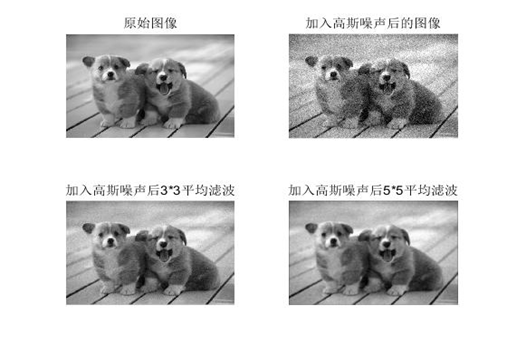加入椒盐噪声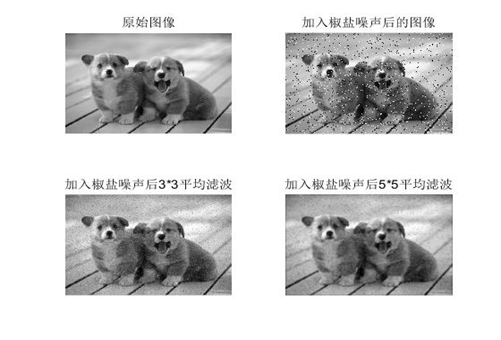平滑中值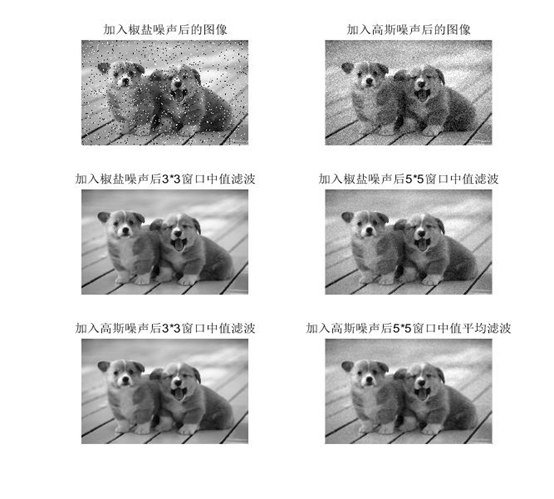### 3 图像处理--锐化

``````//锐化
I=rgb2gray(P);
subplot(221);imshow(I,[]);title('原始图像');
K=histeq(I);
subplot(222);imshow(K);title('由均衡化后的直方图得到的均衡后的图像');
BW=edge(K,'roberts',0.1);
subplot(223);imshow(BW);title('均衡后的图像罗伯茨梯度锐化');
h=[0 -1 0;-1 4 -1;0 -1 0];
J=imfilter(I,h);
subplot(224);imshow(J);title('均衡后的图像拉普拉斯锐化');
``````

### 实验结果